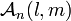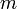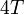VasCalc Results - ChordMod4T

This page documents some computational results obtained by using the ChordsMod4T software component of the VasCalc project. We denote by${\mathcal A}_n(l,m)$ the formal (rational) vector space generated by all chord diagrams with$n$ chords on a skeleton of$l$ lines and$m$ circles, modulo the$4T$ relations. The algorithm is straightforward: first all such diagrams are constructed, then relations are introduced iteratively over all possible terms in a$4T$ relation. See the documentation for further information about the program and how these results were generated.

Some Dimensions of${\mathcal A}_n(l,m)$

The tables below give the dimensions of${\mathcal A}_n(l,m)$ for various values of$l$,$m$ and$n$. Each table corresponds to a fixed value of$n$ - that is, the number of chords.

This is work in progress - these numbers are not reliable yet!!!

Dimensions of chord diagram spaces with 2 chords
Number of Lines Number of Circles
0 1 2 3 4 5 6 7 8 9
0 0 2 8 24 59 125 237 413 674 1044
1 2 8 24 59 125 237 413 674 1044 1550
2 9 25 60 126 238 414 675 1045 1551 2223
3 28 63 129 241 417 678 1048 1554 2226 3097
4 69 135 247 423 684 1054 1560 2232 3103 4209
5 145 257 433 694 1064 1570 2242 3113 4219 5599
6 272 448 709 1079 1585 2257 3128 4234 5614 7310
7 469 730 1100 1606 2278 3149 4255 5635 7331 9388
8 758 1128 1634 2306 3177 4283 5663 7359 9416 11882
9 1164 1670 2342 3213 4319 5699 7395 9452 11918 14844

Dimensions of chord diagram spaces with 3 chords
Number of Lines Number of Circles
0 1 2 3 4 5 6
0 0 3 19 80 270 770 1918
1 3 19 80 270 770 1918 4284
2 23 88 283 789 1944 4318 8803
3 111 321 845 2021 4419 8931 16876
4 394 954 2172 4618 9184 17189
5 1130 2418 4944 9600 17705 31125
Dimensions of chord diagram spaces with 4 chords
Number of Lines Number of Circles
0 1 2 3 4
0 0 6 44 241 1063
1 6 44 241 1063 3930
2 60 283 1160 4126